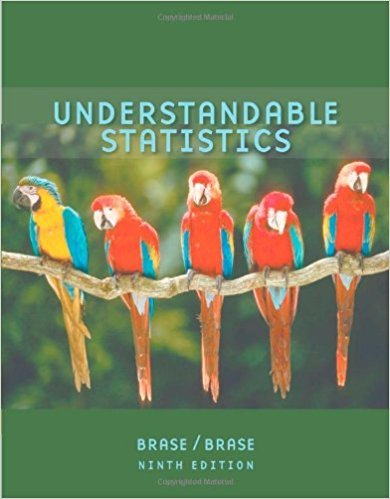×
×

# Solutions for Chapter 11.1: CHI-SQUARE AND F DISTRIBUTIONS## Full solutions for Understandable Statistics | 9th Edition

ISBN: 9780618949922Solutions for Chapter 11.1: CHI-SQUARE AND F DISTRIBUTIONS

Solutions for Chapter 11.1
4 5 0 302 Reviews
26
1
##### ISBN: 9780618949922

This expansive textbook survival guide covers the following chapters and their solutions. This textbook survival guide was created for the textbook: Understandable Statistics, edition: 9. Chapter 11.1: CHI-SQUARE AND F DISTRIBUTIONS includes 15 full step-by-step solutions. Understandable Statistics was written by and is associated to the ISBN: 9780618949922. Since 15 problems in chapter 11.1: CHI-SQUARE AND F DISTRIBUTIONS have been answered, more than 38141 students have viewed full step-by-step solutions from this chapter.

Key Statistics Terms and definitions covered in this textbook
• Alternative hypothesis

In statistical hypothesis testing, this is a hypothesis other than the one that is being tested. The alternative hypothesis contains feasible conditions, whereas the null hypothesis speciies conditions that are under test

• Analytic study

A study in which a sample from a population is used to make inference to a future population. Stability needs to be assumed. See Enumerative study

• Backward elimination

A method of variable selection in regression that begins with all of the candidate regressor variables in the model and eliminates the insigniicant regressors one at a time until only signiicant regressors remain

• Biased estimator

Unbiased estimator.

• C chart

An attribute control chart that plots the total number of defects per unit in a subgroup. Similar to a defects-per-unit or U chart.

• Central composite design (CCD)

A second-order response surface design in k variables consisting of a two-level factorial, 2k axial runs, and one or more center points. The two-level factorial portion of a CCD can be a fractional factorial design when k is large. The CCD is the most widely used design for itting a second-order model.

• Conditional probability distribution

The distribution of a random variable given that the random experiment produces an outcome in an event. The given event might specify values for one or more other random variables

• Conidence level

Another term for the conidence coeficient.

• Contour plot

A two-dimensional graphic used for a bivariate probability density function that displays curves for which the probability density function is constant.

• Control chart

A graphical display used to monitor a process. It usually consists of a horizontal center line corresponding to the in-control value of the parameter that is being monitored and lower and upper control limits. The control limits are determined by statistical criteria and are not arbitrary, nor are they related to speciication limits. If sample points fall within the control limits, the process is said to be in-control, or free from assignable causes. Points beyond the control limits indicate an out-of-control process; that is, assignable causes are likely present. This signals the need to ind and remove the assignable causes.

• Correlation matrix

A square matrix that contains the correlations among a set of random variables, say, XX X 1 2 k , ,…, . The main diagonal elements of the matrix are unity and the off-diagonal elements rij are the correlations between Xi and Xj .

• Critical value(s)

The value of a statistic corresponding to a stated signiicance level as determined from the sampling distribution. For example, if PZ z PZ ( )( .) . ? =? = 0 025 . 1 96 0 025, then z0 025 . = 1 9. 6 is the critical value of z at the 0.025 level of signiicance. Crossed factors. Another name for factors that are arranged in a factorial experiment.

• Cumulative sum control chart (CUSUM)

A control chart in which the point plotted at time t is the sum of the measured deviations from target for all statistics up to time t

• Defects-per-unit control chart

See U chart

• Discrete distribution

A probability distribution for a discrete random variable

• Distribution free method(s)

Any method of inference (hypothesis testing or conidence interval construction) that does not depend on the form of the underlying distribution of the observations. Sometimes called nonparametric method(s).

• Enumerative study

A study in which a sample from a population is used to make inference to the population. See Analytic study

• Experiment

A series of tests in which changes are made to the system under study

• F-test

Any test of signiicance involving the F distribution. The most common F-tests are (1) testing hypotheses about the variances or standard deviations of two independent normal distributions, (2) testing hypotheses about treatment means or variance components in the analysis of variance, and (3) testing signiicance of regression or tests on subsets of parameters in a regression model.

• Goodness of fit

In general, the agreement of a set of observed values and a set of theoretical values that depend on some hypothesis. The term is often used in itting a theoretical distribution to a set of observations.

×